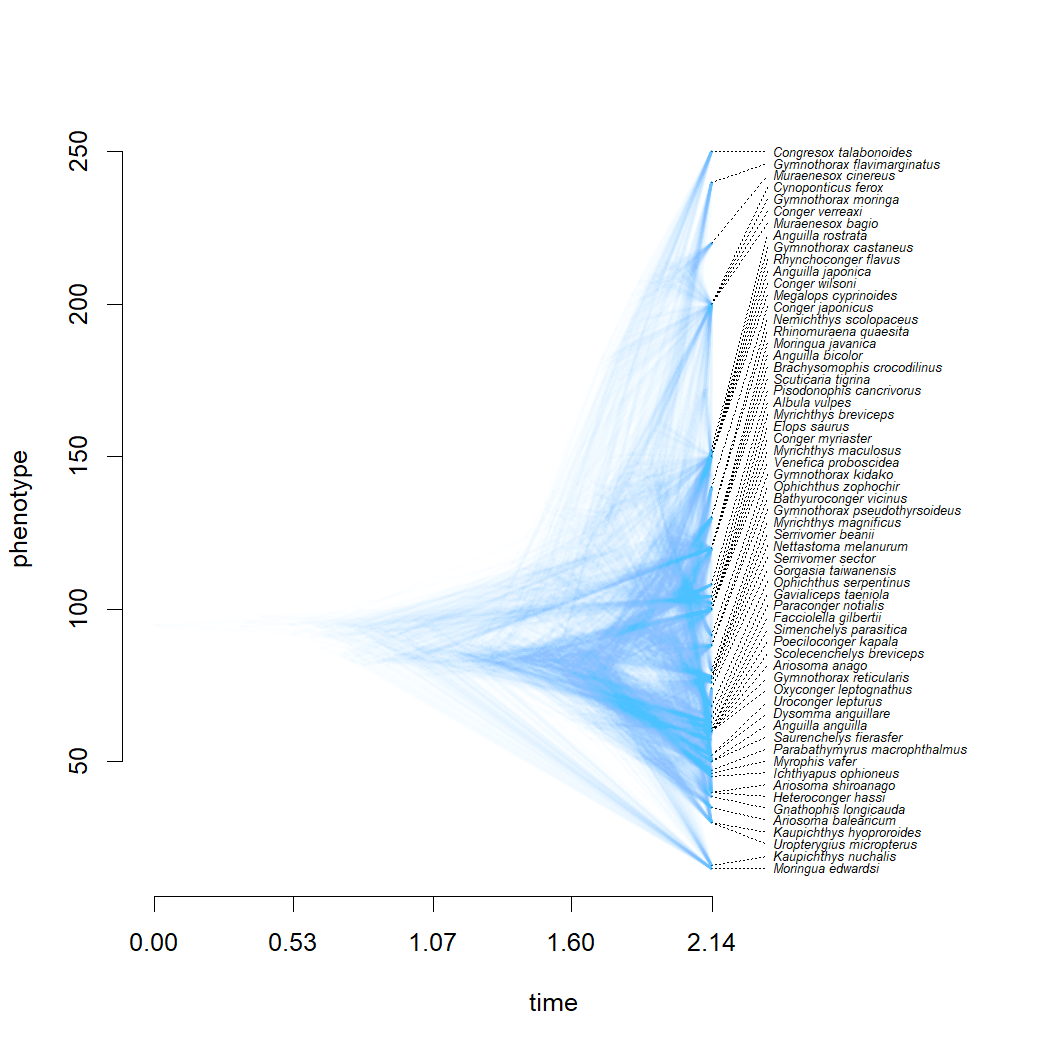## Friday, June 26, 2020

### Creating a traitgram for the set of trees in a sample using phytools

A friend & phytools user contacted me today about plotting a traitgram using the phytools function `phenogram` in which we superimposed the set of trees from a posterior distribution of a Bayesian analysis.

This is not too difficult to do, although there are some complications.

Firstly, the function `phenogram` already includes the option `add=TRUE` which allows you to add one traitgram to an existing traitgram.

The difficult is that trees in a posterior sample will invariably have different depths.

The easiest solution to this is to add a root edge to each tree so that it's total length (including the root edge) is the same, and then convert it to a `"phylo"` object with singleton nodes using `rootedge.to.singleton`.

The only problem with that is that `fastAnc` (the default ancestral state reconstruction method of `phenogram`) uses contrasts internally, and so only works on a bifurcating tree*. (*Actually, it works with multifurcations too - just not with unbranching nodes.)

My work-around for this was to just estimate ancestral states on the tree without the singleton node, and then set the node value before the root edge to be equal to that of the ingroup root. (This is the case for ML under BM anyway.) Then, I painted the crown group and the root edge with different states using `paintSubTree`, and plotted them using `phenogram`.

Here's what the code looks like:

``````library(phytools)
``````
``````## Loading required package: ape
``````
``````## Loading required package: maps
``````
``````Trees<-read.nexus("anguila.nex")
Length<-setNames(Data[,2],rownames(Data))
Trees<-drop.tip.multiPhylo(Trees,
setdiff(Trees[]\$tip.label,
names(Length)))

h<-sapply(Trees,function(x) max(nodeHeights(x)))
ii<-order(h,decreasing=TRUE)
Trees<-Trees[ii]
h<-h[ii]
Trees<-mapply(function(x,r){ x\$root.edge<-r; x },
x=Trees,r=max(h)-h,SIMPLIFY=FALSE)
Trees<-lapply(Trees,rootedge.to.singleton)

for(i in 1:length(Trees)){
a<-fastAnc(collapse.singles(Trees[[i]]),Length)
a<-setNames(c(a,a),1:Trees[[i]]\$Nnode+Ntip(Trees[[i]]))
Trees[[i]]<-paintSubTree(Trees[[i]],node=Ntip(Trees[[i]])+2,
state="1",anc.state="0")
cols<-setNames(c("transparent",make.transparent(palette(),
1/length(Trees))),0:1)
}
``````The posterior for this set of trees is a bit messy. Maybe it would help if I overlay the consensus tree by some measure. Here, I'll use the LS consensus:

``````LStree<-ls.consensus(Trees)
``````
``````## Best Q = 0.742304880125346
``````
``````## Best Q = 0.714112446330444
``````
``````## Best Q = 0.71408931400111
## Best Q = 0.71408931400111
``````
``````## Solution found after 4 set of nearest neighbor interchanges.
``````
``````a<-fastAnc(LStree,Length)
LStree\$root.edge<-max(h)-max(nodeHeights(LStree))
LStree<-rootedge.to.singleton(LStree)
a<-setNames(c(a,a),1:LStree\$Nnode+Ntip(LStree))
par(mar=c(5.1,4.1,2.1,2.1))
color="black",lwd=12,quiet=TRUE,ylab="total length (cm)",
xlab="relative time since the root")